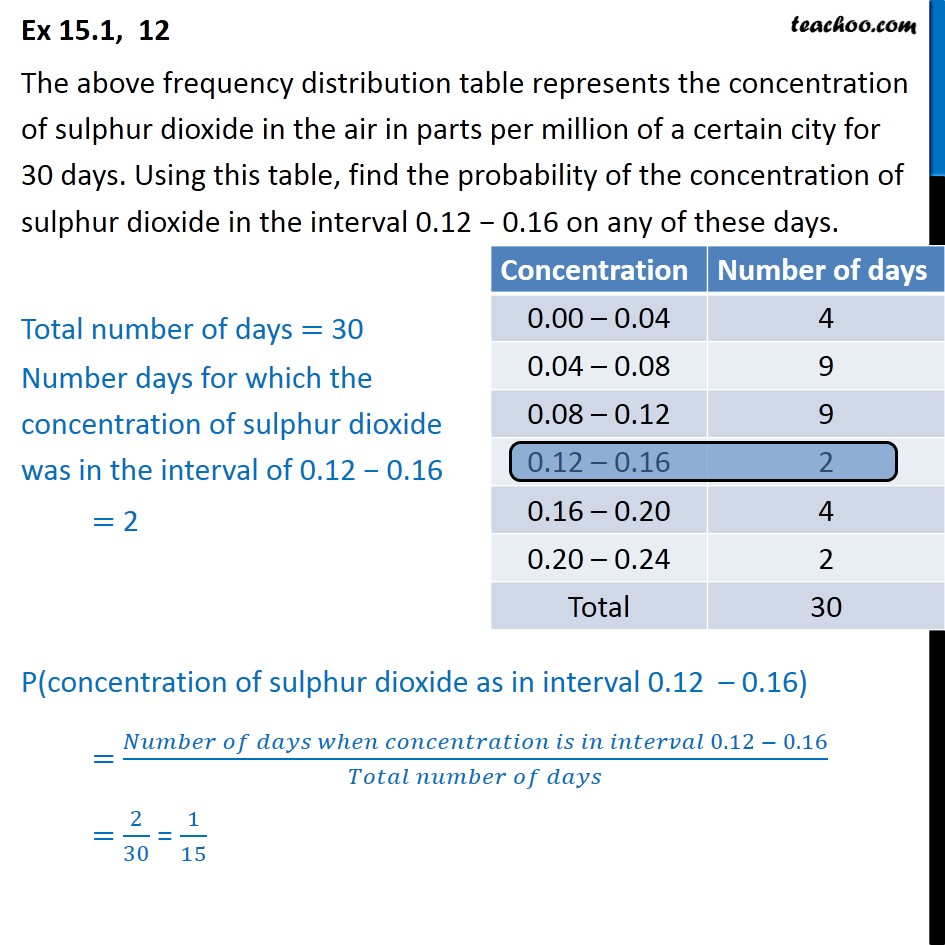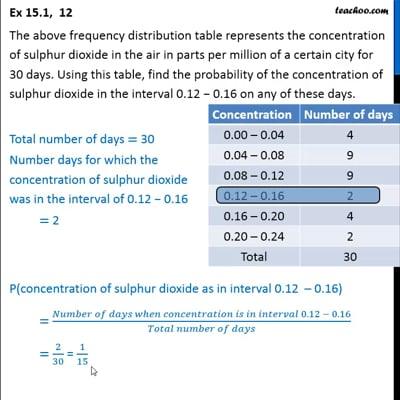Chapter 15 Class 9 Probability

Class 9
Important Questions for Exam - Class 9This video is only available for Teachoo black users

Introducing your new favourite teacher - Teachoo Black, at only ₹83 per month

### Transcript

Ex 15.1, 12 The above frequency distribution table represents the concentration of sulphur dioxide in the air in parts per million of a certain city for 30 days. Using this table, find the probability of the concentration of sulphur dioxide in the interval 0.12 0.16 on any of these days. Total number of days = 30 Number days for which the concentration of sulphur dioxide was in the interval of 0.12 0.16 = 2 P(concentration of sulphur dioxide as in interval 0.12 0.16) = ( 0.12 0.16)/( ) = 2/30 = 1/15